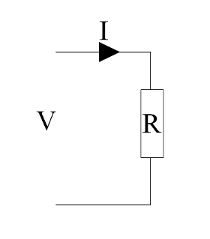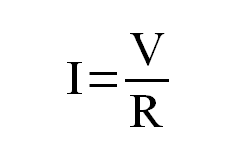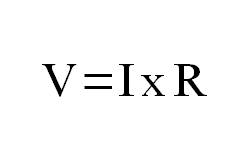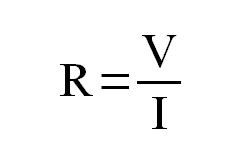Third party cookies may be stored when visiting this site. Please see the cookie information.

# Electronic circuit theory

The electronics course has kept the amount of formula and maths to the minimum. To understand the principles involved and how to design the circuits does need some knowledge of the theory.

The following explains some of the simple theory that is needed for electronic circuit design. Most of this can be applied to real circuits using fairly simple formula. This does not go into the detailed circuit theory that is used for more detailed analysis of circuits as used by SPICE or Matlab etc.

## Ohm's Law

Ohm's law is one of the most important rules applied to electronic circuits. It is used in almost all electronic circuit design. Fortunately it is a very simple law that is easy to apply.

Ohm's law identifies the relationship between voltage, current and resistance in a electrical circuit. The important part is the following equation:Where:
I is the current in amps
V is the voltage in volts
R is the resistance in ohms (Ω)

The above formula provides the current when the voltage and resistance is known, but this can easily be transposed to find the required resistor size if the required current is known or the voltage across a resistor when the current and voltage are known.